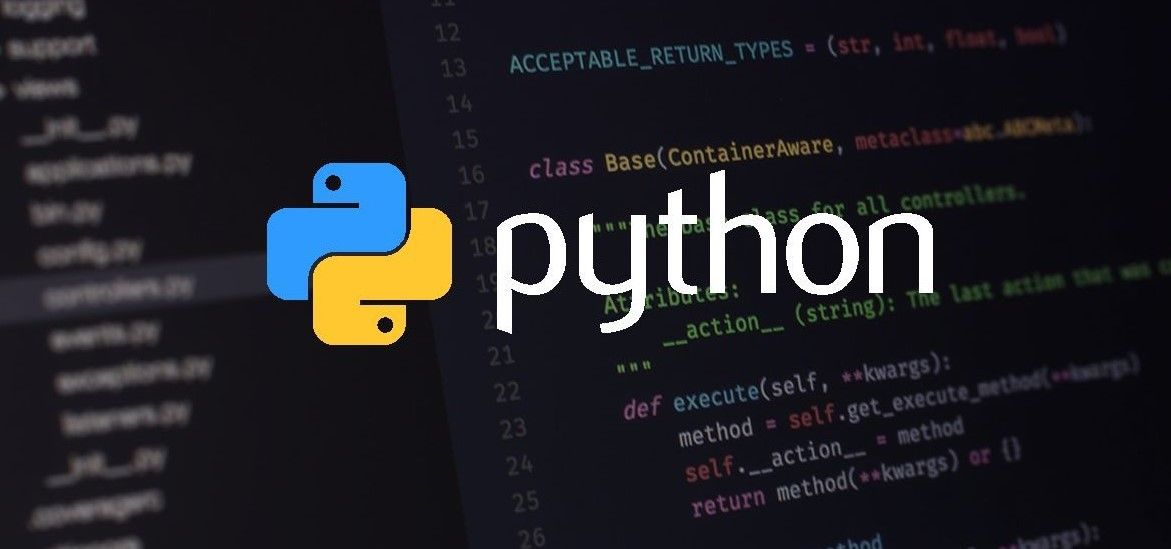### Build your fundamental Python concepts with these 5 Examples

#### Getting started with Python:

• Download and Pre-install Python and PyCharm (Free, open-source) to practice the examples below.
To create a new project in PyCharm - Right Click 'new' on your Project Folder of the left navbar you created. Next, give your file a name and click 'ok'. To run your file, click 'run' located at the top menu bar.Example 1: Print "Hello World"

print("Hello World")

Output: Hello World

Example 2: Drawing a Shape

print("   /|")
print("  /  |")
print(" /   |")
print("/__ |")

Output:
/|
/  |
/   |
/__ |

*In Python, order of the instruction matters.

Example 3: Variable and Data Types

print("There once was a man named Bob")
print("He was 70 years old.")
print("He really liked the name Bob")
print("but didn't like being 70.")

Output:

There once was a man named Bob
He was 70 years old.
He really liked the name Bob
but didn't like being 70.

character_name = "Bob"
character_age = "70"
is_male = True
print("There once was a man named " + character_name +", ")
print("He was " + character_name + " years old. ")
print("He really liked the name" + character_name +", ")
print("but didn't like being " + character_age +".")

Output:

There once was a man named Bob,
He was Bob years old.
He really liked the nameBob,
but didn't like being 70.

Example 4: Working with Strings

print("Semiconductor\nBlog")

Output:
Semiconductor
Blog

phrase = "Semiconductor Blog"
print(phrase)

Output:
Semiconductor Blog

Concatenation - Process of taking a string and appending another string upon to it.

phrase = "Semiconductor Blog"
print(phrase + " is cool")

Output:
Semiconductor Blog is cool

phrase = "Semiconductor Blog"
print(phrase.lower())

Output:
semiconductor blog

phrase = "Semiconductor Blog"
print(phrase.upper())

Output:
SEMICONDUCTOR BLOG

phrase = "Semiconductor Blog"
print(phrase.isupper())

Output:
False

phrase = "Semiconductor Blog"
print(phrase.upper().isupper())

Output:
True

phrase = "Semiconductor Blog"
print(len(phrase))

Output:
18

phrase = "Semiconductor Blog"
0123...................
print(phrase)

Output:
S

phrase = "Semiconductor Blog"
print(phrase)

Output:
i

phrase = "Semiconductor Blog"
print(phrase.index("i"))

Output: index function > passing a parameter
3

phrase = "Semiconductor Blog"
print(phrase.index("Blog"))

Output: index function > passing a parameter
15

phrase = "Semiconductor Blog"
print(phrase.index("x"))

Output: index function > passing a parameter
Value Error

phrase = "Semiconductor Blog"
print(phrase.replace("Semiconductor", "SemiSaga"))

Output:
SemiSaga Blog

Example 5: Working with Numbers

print(2)

Output:
2

print(2.32)

Output:
2.32

print(-2)

Output:
-2

print(2 + 4.5)

Output:
6.5

print(3 * 4)

Output:
12

print(3 * 4 + 5)

Output:
17

print(3 * (4 + 5))

Output:
27

print(10 % 3)

Output: % is the modulus => remainder
1

my_num = 5
print(my_num)

Output:
5

my_num = 5
print(str(my_num) + " my favorite number")

Output:
5 my favorite number

my_num = -5
print(abs(my_num))

Output:
5

my_num = 5
print(pow(4, 6))

Output:
4096

my_num = -5
print(max(4, 6))

Output:
6

my_num = 5
print(min(4, 6))

Output:
4

my_num = -5
print(round(3.2))

Output:
3

from math import *
my_num = -5
print(floor(3.7))

Output:
3

from math import *
my_num = -5
print(sqrt(36))

Output:
6.0

1.